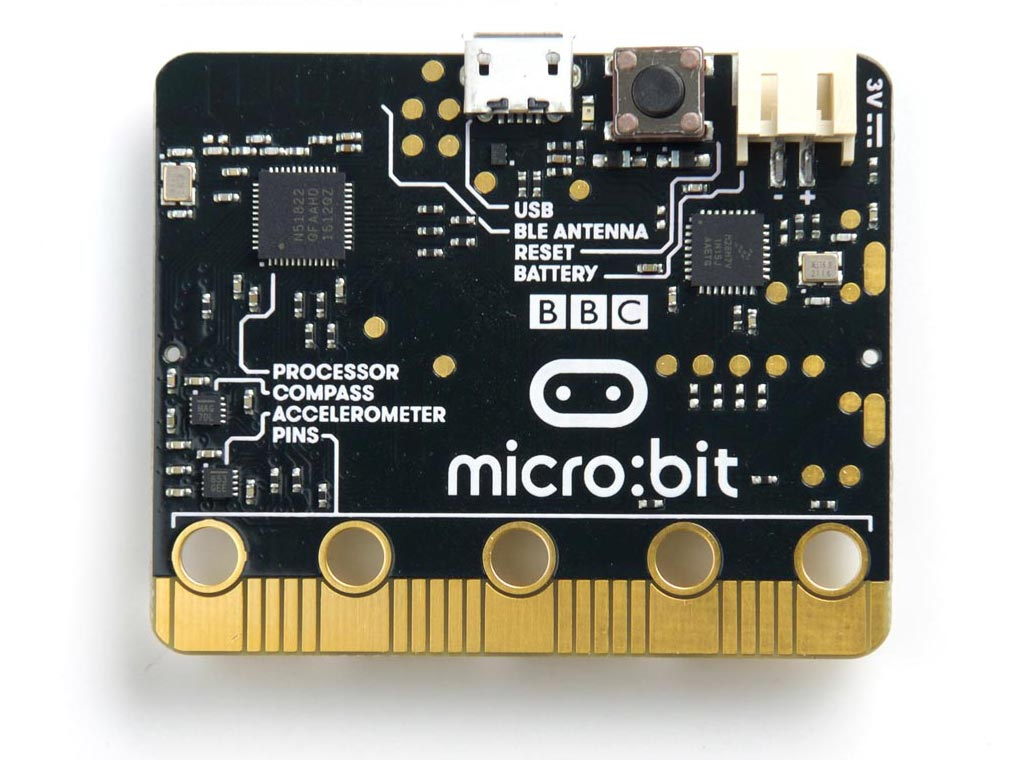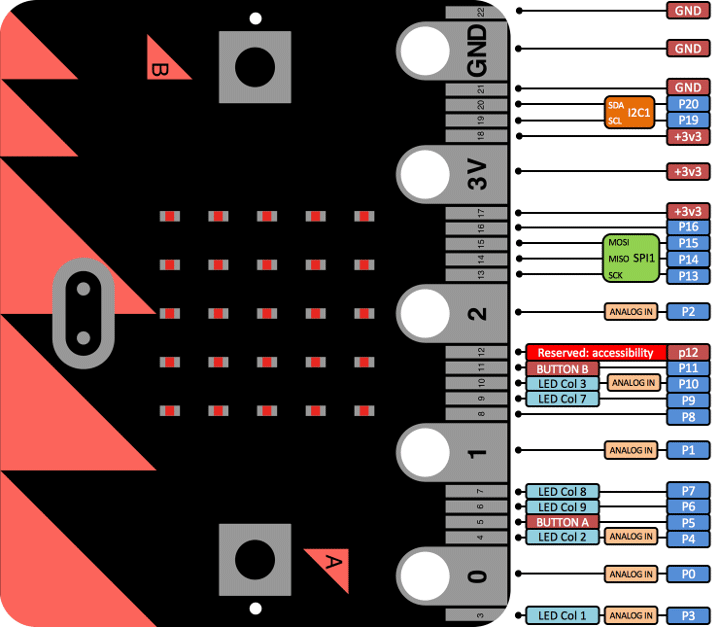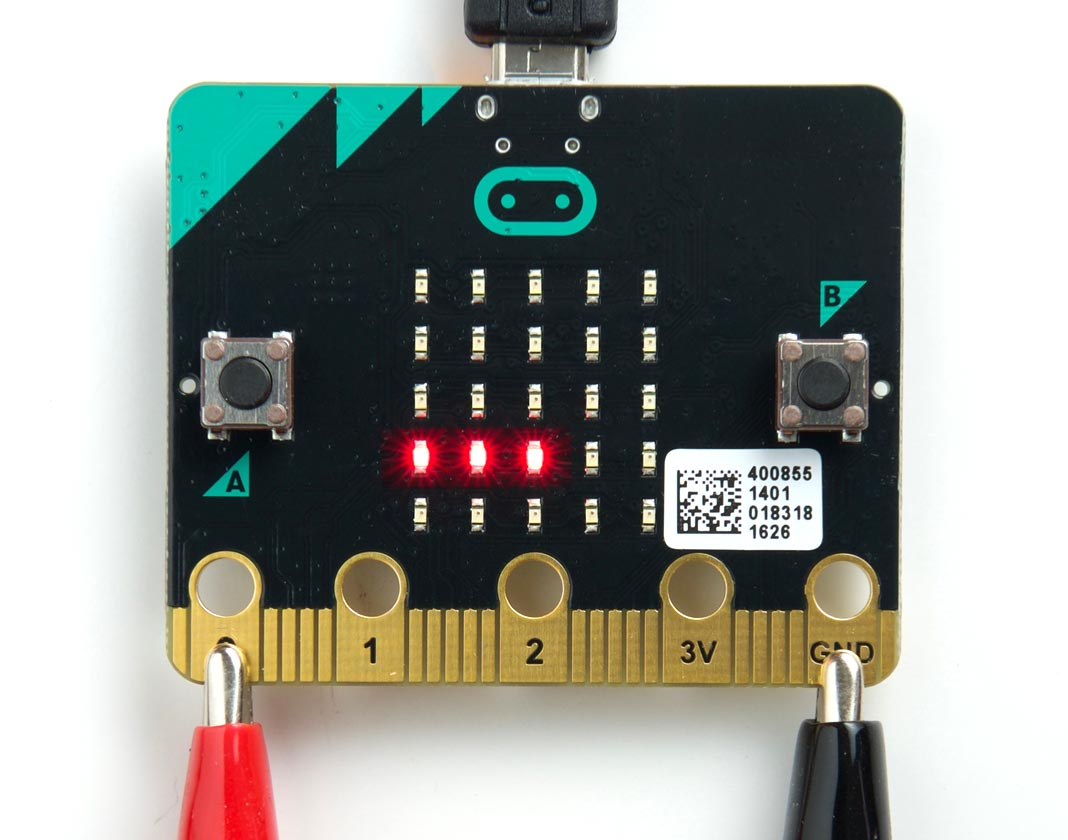## BBC Micro Bit

The ARM version of uLisp now runs on the BBC Micro Bit, the low-cost controller board pioneered by the BBC in the UK.

The BBC Micro Bit is based on a Nordic Semiconductor nRF51822 ARM Cortex-M0 microcontroller. It runs at 16 MHz and provides 256 Kbytes of flash program memory and 16 Kbytes of RAM:### Pin connections

The BBC Micro Bit provides 19 digital input/output pins, 6 analogue inputs, and 19 analogue outputs. They are accessible from the edge connector as follows:The board also includes two pushbuttons and a matrix of 5 x 5 multiplexed LEDs. See Example programs below for examples of using these from uLisp.

### Installing uLisp on the BBC Micro Bit

You can install the ARM version of uLisp on a BBC Micro Bit using the Arduino IDE as follows:

• In the Arduino IDE open the Preferences dialog box, and add the following URL to Additional Board Manager URLs:
`https://sandeepmistry.github.io/arduino-nRF5/package_nRF5_boards_index.json`
• Open the Boards Manager… from the Board item on the Tools menu, search for nRF5, and install Nordic Semiconductor nRF5 Boards by Sandeep Mistry.
• Connect the BBC Micro Bit to your computer using a USB cable.
• Select BBC micro:bit from the Board menu, set Softdevice to None, and set Port to the USB port.

You should now be able to upload uLisp to the BBC Micro Bit.

Follow the instructions in Using uLisp to interact with uLisp on the BBC Micro Bit.

### Example programs

#### Buttons

The reverse side of the BBC Micro Bit provides two pushbuttons on pins 5 and 11. You can read these with the following program:

```(defun buttons ()
(pinmode 5 nil)
(pinmode 11 nil)
(loop
(print
(delay 1000)))```

Run it by typing:

`(buttons)`

#### LEDs

The reverse of the BBC Micro Bit also provides a matrix of 5 x 5 LEDs. They are connected in a totally illogical arrangement of nine columns and three rows, and to light any individual LED you need to take the column low and the row high corresponding to that LED.

The following table shows the (row, column) for each LED:

 (26, 3) (27, 23) (26, 4) (27, 24) (26, 10) (28, 23) (28, 24) (28, 25) (28, 9) (28, 7) (27, 4) (26, 6) (27, 10) (28, 6) (27, 3) (26, 7) (26, 9) (26, 25) (26, 24) (26, 23) (28, 10) (27, 9) (28, 3) (27, 25) (28, 4)

This program flashes the LED at (row, column):

```(defun b (row col)
(let (x)
(pinmode row t)
(pinmode col t)
(digitalwrite row t)
(loop
(digitalwrite col x)
(delay 1000)
(setq x (not x)))))```

For example, to flash the centre LED run:

`(b 27 10)`

#### Analogue outputs

Any pin can also be used as an 8-bit analogue output, so you can pulsate the centre LED slowly on and off with the program:

```(defun pulse ()
(let (down)
(pinmode 27 t)
(digitalwrite 27 t)
(loop
(dotimes (x 256)
(delay 5)
(analogwrite 10 (if down (- 255 x) x)))
(setq down (not down)))))```

Run it by typing:

`(pulse)`

Exit from any program by entering ~.

#### Analogue inputs

The BBC Micro Bit provides six 10-bit analogue inputs, three of which are available on the edge connector pins labelled 0, 1, and 2. The following example program reads the analogue input on pin 0 and displays the value as a bar of LEDs, using the fourth row of LEDs on the matrix:The program takes advantage of the fact that this row of LEDs share the same row number. Here's the program:

```(defun meter ()
(let ((col '(7 9 25 24 23))
(row 26))
(pinmode row t)
(digitalwrite row t)
(mapc (lambda (x) (pinmode x t)) col)
(loop
(dotimes (n 5)
(digitalwrite
(nth n col)
(<
`(meter)`